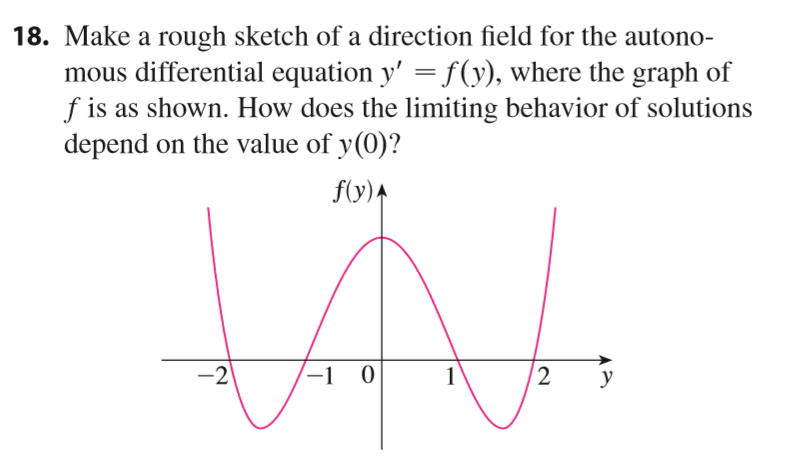# 18. Make a rough sketch of a direction field for the autonomous differential equation y' fy), where the graph off is as shown. How does the limiting behavior of solutionsdepend on the value of y(0)?f(y)A2-1 0-21

Question
332 views

How does the limiting behavior of solutions depend on the value of y(0)?help_outlineImage Transcriptionclose18. Make a rough sketch of a direction field for the autono mous differential equation y' fy), where the graph of f is as shown. How does the limiting behavior of solutions depend on the value of y(0)? f(y)A 2 -1 0 -2 1 fullscreen
check_circle

star
star
star
star
star
1 Rating
Step 1

Given autonomus differential equation:

Graph of function f is given.
We need to show the the limiting behavior of solution depend on the value of y(0)

Step 2

Since given graph is symmetric about the vertical y -axis. So, f(-y) = f(y). It means slope is not change the reflection about the horizontal axis. Point (x,y) and (x, -y) are same.

Since value of function is 0 :

the slopes are horizontal on these four lines, giving rise to four constant solutions.

Given graph\'s slope doesn\'t depend on x, it means direction field doesn\'t change under the horizontal shift.

Step 3

Given graph is positive for interval:

In these regions direction fi...

### Want to see the full answer?

See Solution

#### Want to see this answer and more?

Solutions are written by subject experts who are available 24/7. Questions are typically answered within 1 hour.*

See Solution
*Response times may vary by subject and question.
Tagged in

### Differential Equations